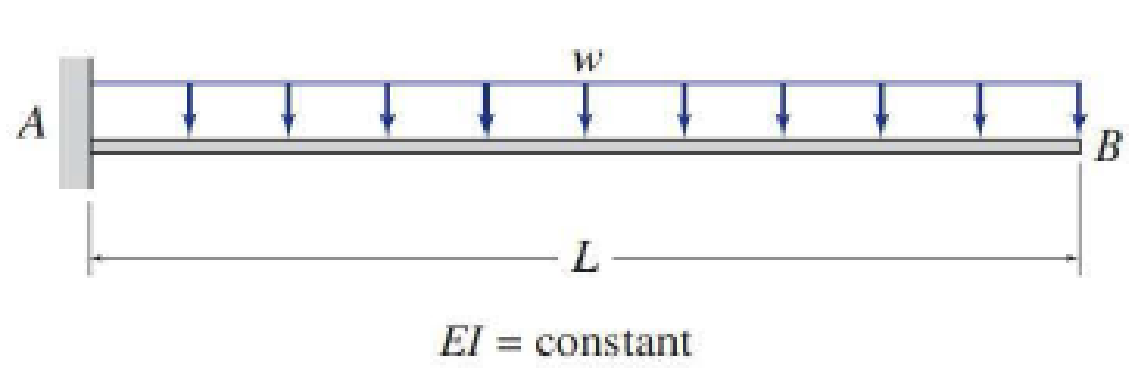# Determine the slope and deflection at point B of the beam shown by the moment-area method. FIG. P6.10, P6.36

#### Solutions

Chapter
Section
Chapter 6, Problem 10P
Textbook Problem
549 views

## Determine the slope and deflection at point B of the beam shown by the moment-area method.FIG. P6.10, P6.36

To determine

Find the slope θB and deflection ΔB at point B of the given beam using the moment-area method.

### Explanation of Solution

Calculation:

Consider the flexural rigidity EI of the beam is constant

Show the given beam as in Figure (1).

Consider clockwise as negative and counterclockwise as positive.

Determine the bending moment at point A using the relation;

MA=w×L×L2

Here, w is the uniformly distributed load and l is the distance.

MA=wL22

Show the M/EI diagram for the given beam as in Figure (2).

Elastic curve:

The sign of M/EI diagram is negative, therefore, the beam bends downward. The support A of the given beam is fixed and the slope at A is zero. Therefore, the tangent to the elastic curve at A is horizontal.

Show the elastic curve diagram as in Figure (3).

The slope at point B can be calculated by evaluating the change in slope between A and B.

Express the change in slope using the first moment-area theorem as follows:

θB=θBA=AreaoftheM/EIbetweenAandB=13×b×h

Here, b is the width and h is the height of the triangle.

Substitute L for b and wl22EI for h.

θB=θBA=13×L×(wL22EI)=wL36EI

Hence, the slope at point B is wL36EI_

### Still sussing out bartleby?

Check out a sample textbook solution.

See a sample solution

#### The Solution to Your Study Problems

Bartleby provides explanations to thousands of textbook problems written by our experts, many with advanced degrees!

Get Started

Find more solutions based on key concepts
Give examples of Excels logical functions and relational operators.

Engineering Fundamentals: An Introduction to Engineering (MindTap Course List)

List the sections of a system design specification, and describe the contents.

Systems Analysis and Design (Shelly Cashman Series) (MindTap Course List)

What is an advantage of using locking pliers?

Precision Machining Technology (MindTap Course List)

Which security protocols are used to protect e-mail?

Principles of Information Security (MindTap Course List)

Describe the role of a CIO within an organization.

Principles of Information Systems (MindTap Course List)

Describe the activities of the analysis phase. List the three activities of the detailed analysis phase.

Enhanced Discovering Computers 2017 (Shelly Cashman Series) (MindTap Course List)

What are two ways to provide input for the speedometer?

Automotive Technology: A Systems Approach (MindTap Course List)

If your motherboard supports ECC DDR3 memory, can you substitute non-ECC DDR3 memory?

A+ Guide to Hardware (Standalone Book) (MindTap Course List)